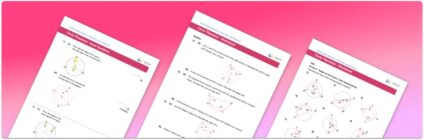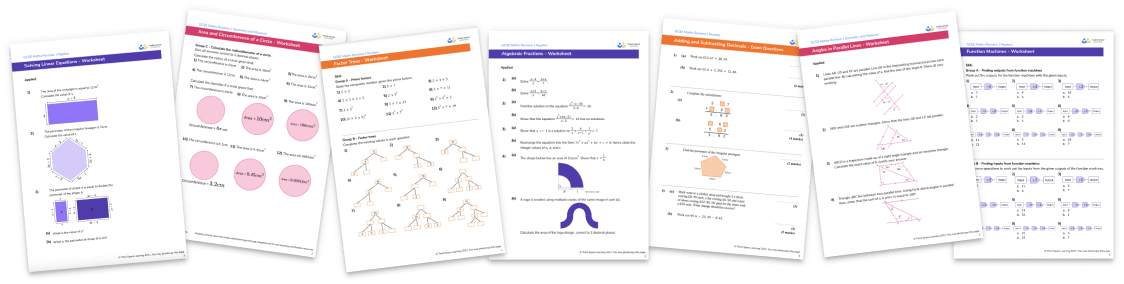# Circle Theorems Worksheet• Section 1 of the circle theorems  worksheet contains 36 skills-based circle theorems questions, in 3 groups to support differentiation
• Section 2 contains 4 applied circle theorems questions with a mix of worded problems and deeper problem solving questions
• Section 3 contains 4 foundation and higher level GCSE exam style questions
• Answers and a mark scheme for all questions are provided
• Questions follow variation theory with plenty of opportunities for students to work independently at their own level
• All questions created by fully qualified expert secondary maths teachers
• Suitable for GCSE, IGCSE and KS4 maths revision for AQA, OCR and Edexcel exam boards

• This field is for validation purposes and should be left unchanged.

You can unsubscribe at any time (each email we send will contain an easy way to unsubscribe). To find out more about how we use your data, see our privacy policy.

### Circle Theorems at a glance

Circle theorems are a set of angle properties that apply within circles. We can use the circle theorems to work out missing angles.

To access circle theorems we need to be confident with the names of the key parts of a circle including the centre of the circle, the diameter of the circle, the radius of the circle, a tangent to the circle, a segment of the circle, a sector of the circle and a chord within the circle.

We also need to be confident at labelling angles using three letter notation. For example, angle BCD is the angle created at C when travelling from B to C to D. The centre of a circle is often labelled O, in which case the angle BOD would be the angle at the centre O when travelling from B to O to D.

The circle theorems are:

• The angle at the centre is twice the angle at the circumference of a circle
• Angles in the same segment are equal
• Opposite angles in a cyclic quadrilateral are equal
• The angle at the circumference of the circle in a semicircle is a right angle
• The angle between a tangent and a radius is a right angle
• Two tangents which meet at a point are equal in length
• The alternate segment theorem

Looking forward, students can then progress to additional and other geometry worksheets, for example an angles in polygons worksheet or area and circumference of a circle worksheet.For more teaching and learning support on Geometry our GCSE maths lessons provide step by step support for all GCSE maths concepts.

## Do you have KS4 students who need more focused attention to succeed at GCSE?There will be students in your class who require individual attention to help them succeed in their maths GCSEs. In a class of 30, it’s not always easy to provide.

Help your students feel confident with exam-style questions and the strategies they’ll need to answer them correctly with our dedicated GCSE maths revision programme.

Lessons are selected to provide support where each student needs it most, and specially-trained GCSE maths tutors adapt the pitch and pace of each lesson. This ensures a personalised revision programme that raises grades and boosts confidence.

Find out more